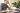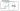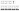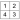# Aptitude Test – Practice Sample QuestionsHere are a few sample questions for you to get a feel for what pre-employment aptitude tests are all about. Keep in mind though, each test is different, and you should practice as much as you can with full-length mock tests, of the specific test you are about to take. Studies have proven that practicing does improve your average score significantly. So take your time, find a quiet spot, and it’s game on!

### Question 1 – Verbal Ability

POETRY is to LITERATURE as…

A. RHYTHM is SYNTAX
C. LYRICS is to PROSE
D. SCULPTURE is to VISUAL ART
E. ARTIST is to CRAFTSMAN

The analogy underlying this question is ‘A is a type of B’.
The example – poetry is a type of literature.
The correct option – sculpture is a type of visual arts.

A tip for a quick solution

Always try to form the simplest underlying structure of the analogy. Try adding additional properties to it only if more than a single answer-choice manifests the same structure.

### Question 2 – Verbal Ability

Assume the first two statements are true. Is the conclusion: 1) True, 2) False, or 3) Uncertain based on the information provided?

Statement 1 – All the trees do not have leaves which are not green.
Statement 2 – The Gwendoline has green leaves.
Conclusion – The Gwendoline is a tree.

The correct answer is (3) – the conclusion is uncertain

This question presents a rule that applies to trees and additional information regarding the Gwendoline.

Statement A (rule): Trees –> have either green, yellow, or red leaves
Statement B (information): The Gwendoline has green leaves.

According to statement A, we can conclude that if a tree has leaves, then these leaves are definitely green. However, we cannot conclude that anything with green leaves is a tree. There can be other things with green leaves which are not trees. Therefore, although statement B tells us that the Gwendoline has green leaves, we cannot conclude that the Gwendoline is a tree.

A tip for a quick solution

You can draw on a piece of paper or in your mind diagrams that depict the information presented in the statements. These diagrams make it easier to understand whether a conclusion is absolutely true/false or if there are also additional possible conclusions.

The diagram below depicts the statement above and points at two possible locations for the Gwendoline, either in the group of the trees or outside of that group. Notice that the diagram even demonstrates that there can be trees with no leaves.### Question 3 – Numerical Ability

UGTA processed 200 student applications during September. If the university processed 45% more applications in the following month, how many applications did it process during October?

A. 245
B. 285
C. 290
D. 298
E. 310

The basic formula to work percentages is (basic value/100) X value of increment/deduction. In our case, the basic value is 200 applications. The required increment is 45%, thus the calculation is: 200/100 X 45 = 2 X 45 = 90.

Therefore, the number of applications processed during October = basic value + 45% = 200 + 90 = 290.

A Tip for a quick solution

instead of calculating 45% directly, you may find it easier to calculate it as 50% minus 5%.

### Question 4 – Numerical Ability

What is the arithmetic mean of the following group of numbers: 3, 5, 16, 17, and 30?

A. 14.2
B. 13.8
C. 15.25
D. 14.6
E. 15.2

Explanation: Arithmetic mean (or simply the average) is the sum of all values divided by the number of values.

Sum of values: 5 +16 +17 + 3 + 30 = 71
Number of values: 5
The average: 71/5 = 14.2

A tip for a quick solution

While performing a basic operation (multiplication, division) with 2-digit numbers, try performing the operation separately on a single digit at first. This action alone may eliminate most of the answer-choices and sometimes even all the options but the correct one.

In this case, the units digit of 71 is divided by five and equals 0.2. This observation eliminates all answer-choices but two. Understanding that 70/5 = 14 eliminates all answer choices but the correct one.

### Question 5 – Non-Verbal Ability

Which of the following boxes should replace the question mark (?) to complete the pattern?Explanation: Each element in the sequence contains four inner shapes. Imagine that each element is divided into four quarters as described in this chart:In each step, each of the four elements moves to an adjacent quarter clockwise (1→2; 2→3; 3→4; 4→1).

A tip for a quick solution

Arrows might be very distracting. One tends to assume that their direction must be significant for the
solution, but this is not always the case. In this question, each arrow is a simple inner shape.

### Question 6 – Non-Verbal Ability

Which of the following does not follow the same logic as the rest?All the elements but C contain four different types of shapes. Element C contains five different types of shapes.

A tip for a quick solution

When you encounter a question with many different inner shapes, you should mainly consider these three elements:

1. The type of shapes
2. The number of items
3. The location of specific shapes